Home > CALC > Chapter 8 > Lesson 8.1.1 > Problem8-6

8-6.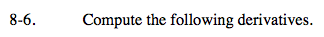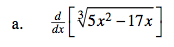Before differentiating, rewrite the expression with an exponent.

Take the derivative using the Chain Rule.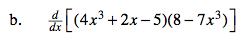You can expand the polynomial expression before differentiating or you can compute the derivative using the Product Rule.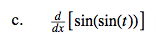Chain Rule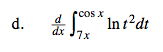≠ ln(cos2(x)) – ln(49x2)

The Fundamental Theorem of Calculus, part 1 states that the derivative of an INDEFINITE integral is the original function. But this is a DEFINITE integral.

= −sin(x)ln(cos2(x)) − 7ln(49x2)